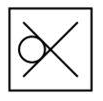Question 4

# From the given answer figures, select the one in which the question figure is hidden/embedded.Solution

There is only one diagonal in the last three figures, and we need two to make the 'X' figure. Sp, if we rotate the question figure $$90^\circ$$ clockwise, we will get the image embedded in first figure.

=> Ans - (A)